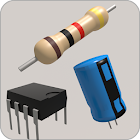Electronics Toolkit Pro

• SEARCH TYPE

All Android applications categories

All Android games categoriesElectronics Toolkit Pro

by: 104 9.6

9.6 Users
rating

Screenshots

Description

Electronics Toolkit Pro is an ad-free version of Electronics Toolkit. It is a helpful app with dozens of tools, calculators and references made for electronic engineers, students and hobbyists.

Extras:
• More Arduino boards
• Unit selector for each input
• More features comming soon

Calculators:
• Resistor color codes - calculate the resistance of resistors by selecting the colors of the bands

• SMD resistor codes - calculate the resistance of SMD resistors by entering the number

• LED resistor calculator - calculate the needed resistance to connect en LED to a power source

• Parallel resistors - calculate the resistance of resistors in parallel

• Voltage divider - calculate the output voltage of a voltage divider

• Series resistors - calculate the resistance of resistors in series

• Ohm's law - calculate the voltage, current of resistance by entering the other two

• Capacitance calculator - calculate the capacitance, voltage or charge by entering the other two

• Battery discharge - calculate the time it takes to discharge a battery

• Inductor color codes - calculate the inductance of inductors by selecting the colors of the bands

• Parallel capacitors - calculate the capacitance of capacitors in parallel

• Series capacitors - calculate the capacitance of capacitors in series

• Unit converter - unit converter for length, temperature, area, volume, weight, time, angle, power and base

• Op-amp calculator - calculate the output voltage of non-inverting, inverting, summing and differential opamps

• Wheatstone bridge - calculate the resistance of one resistor in a balanced bridge or calculate the output voltage

• Inductor codes - calculate the inductance of inductors by entering the number

• Capacitor codes - calculate the capacitance of capacitors by entering the number

• DAC and ADC calculator - calculate the output of digital-analog and analog-digital converters

• Wavelength frequency calculator - calculate the frequency or wavelength of a wave

• SI prefixes - convert numbers with SI prefixes

• Capacitor energy - calculate the energy in an capacitor

• Slew rate calculator - calculate the slew rate

• Star delta transformation - calculate the resistors in a star delta transformation

• Zener calculator - calculate the resistance of the resistor and voltage of the zener

• Air core inductor calculator - calculate the inductance and wire length of an air core inductor

• 555 timer calculator - calculate the frequency, period, duty cycle, high time and low time of a popular 555 timer circuit

• Plate capacitor calculator - calculate the capacity of a plate capacitor

• Resistance to color code calculator - calculate the colors on the resistor by entering the resistance

• LM317 - calculate the output voltage of an LM317

• Low pass filters

• Wire resistance - calculate the resistance of electrical wire

• RMS voltage

• Decibel calculator

•Reactance

Tables:
• Logic gates - truth table of the 7 logic gates with interactive buttons

• 7-segment display - interactive display that you can change by clicking on one of the segments or by clicking on a button to show a hexadecimal character

• ASCII - decimal, hexadecimal, binary, octal and char ASCII table

• Resistivity - table with the resistivity of common metals at 293K

• Arduino pinout

• Pinout diagrams of 4000 and 7400 series ICs

Other:
• Bluetooth - connect to a bluetooth module like the HC-05 to talk with an arduino or other microcontroller with the terminal, button and slider modes

Users review

from 104 reviews

"Awesome"

9.6